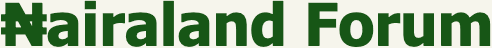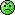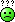Welcome, Guest: Join Nairaland / LOGIN! / Trending / Recent / New
Stats: 2,352,651 members, 5,217,611 topics. Date: Saturday, 19 October 2019 at 08:39 PM

Chemistry! Help A Brother To Solve These Questions - Programming - Nairaland

(1) (Reply) (Go Down)

 Chemistry! Help A Brother To Solve These Questions by LaiveNg: 8:02pm On Feb 15, 2018 Hello, bosses, fellow developers and programmers, i am aware this isn't the right forum to post this thread; i have been banned from posting on the NL Education forum. I have the following questions from my pre-lab manual. If you can help me with solutions, i will be ever grateful..1. You want to prepare a 50cm3 of 0.1M NaOH from exactly 50% (wt/wt) NaOH solution. What volume of the 50% solution is required? Re: Chemistry! Help A Brother To Solve These Questions by LaiveNg: 8:08pm On Feb 15, 2018 2. An aqueous sample is analyzed and found to contain 4% NaOH:.a. Calculate the molar conc of the solution, assuming the density of water is 1.00g/cm3.b. If the solution is neutralized with 1.0M H2SO4, what volume of the acid will be needed? Re: Chemistry! Help A Brother To Solve These Questions by iHart(m): 8:22pm On Feb 15, 2018 1. measure ~ 0.19 mL into a 50 mL volumetric flask/container and make it up to the 50 mL mark. ...is it correct? Re: Chemistry! Help A Brother To Solve These Questions by charliboy654(m): 8:30pm On Feb 15, 2018 I did chemistry like 6yrs ago, can't remember shît about it again. Re: Chemistry! Help A Brother To Solve These Questions by Nobody: 8:40pm On Feb 15, 2018 LaiveNg:2. An aqueous sample is analyzed and found to contain 4% NaOH:.a. Calculate the molar conc of the solution, assuming the density of water is 1.00g/cm3.b. If the solution is neutralized with 1.0M H2SO4, what volume of the acid will be needed?I hated this topic1 Like Re: Chemistry! Help A Brother To Solve These Questions by LaiveNg: 8:52pm On Feb 15, 2018 iHart:1. measure ~ 0.19 mL into a 50 mL volumetric flask/container and make it up to the 50 mL mark. ...is it correct?Thanks, but i think the questions require a bit math to buttress the significance of the figures. Re: Chemistry! Help A Brother To Solve These Questions by iHart(m): 10:03pm On Feb 15, 2018 LaiveNg:Thanks, but i think the questions require a bit math to buttress the significance of the figures. but the question was to state the volume and not to show working. so that's why I just gave you that... Re: Chemistry! Help A Brother To Solve These Questions by iHart(m): 10:10pm On Feb 15, 2018 LaiveNg:2. An aqueous sample is analyzed and found to contain 4% NaOH:.a. Calculate the molar conc of the solution, assuming the density of water is 1.00g/cm3.b. If the solution is neutralized with 1.0M H2SO4, what volume of the acid will be needed?a. 1.0Mb. 50 mL (assuming the basis of the % is same with the volume of base) Re: Chemistry! Help A Brother To Solve These Questions by horlahwaley(m): 10:14pm On Feb 15, 2018 1. you need to convert 50%(wt / wt ) to wt/ v and den find the molar conc.note: 50g of water will give 50ml/cm3 of water since the density of water is 1.0g/cm3note also: 1ml =1cm3to do that (50g of naoh ÷ 50cm3 of diluent/h20) ×100cm3dat should give u 1g/100cm3 which is the mass conc in 100cm3but in 1000cm3 the solute will be 10gtherforefinal mass conc is 10g/1000cm3 or 10g/dm3now convert the mass conc to molar conc molar conc= mass conc/ molar mass of. naoh10/40= 0.25Mused this formular RV/OR= required conc 0.1 MV= required volume 50ml0= original conc 0.25Molar(0.1×50)/0.25=20mltherfore 20ml of 50%w/w naoh is needed and made itnup with 80ml.of diluent. Re: Chemistry! Help A Brother To Solve These Questions by LaiveNg: 10:22pm On Feb 15, 2018 horlahwaley:1. you need to convert 50%(wt / wt ) to wt/ v and den find the molar conc.note: 50g of water will give 50ml/cm3 of water since the density of water is 1.0g/cm3note also: 1ml =1cm3to do that (50g of naoh ÷ 50cm3 of diluent/h20) ×100cm3dat should give u 1g/100cm3 which is the mass conc in 100cm3but in 1000cm3 the solute will be 10gtherforefinal mass conc is 10g/1000cm3 or 10g/dm3now convert the mass conc to molar conc molar conc= mass conc/ molar mass of. naoh10/40= 0.25Mused this formular RV/OR= required conc 0.1 MV= required volume 50ml0= original conc 0.25Molar(0.1×50)/0.25=20mltherfore 20ml of 50%w/w naoh is needed and made itnup with 80ml.of diluent. WOW!!! Thanks a bunch! You're worth it. Can i please post moreRe: Chemistry! Help A Brother To Solve These Questions by iHart(m): 11:14pm On Feb 15, 2018 LaiveNg:Hello, bosses, fellow developers and programmers, i am aware this isn't the right forum to post this thread; i have been banned from posting on the NL Education forum. I have the following questions from my pre-lab manual. If you can help me with solutions, i will be ever grateful..1. You want to prepare a 50cm3 of 0.1M NaOH from exactly 50% (wt/wt) NaOH solution. What volume of the 50% solution is required?I think the answer should rather be 0.4 mL. First of all what is the Molar conc of 50% wt/wt of NaOH?50% wt/wt means 50g NaOH/100g water.If we assume density of water is 1...then you have 50g NaOH /100mL water.thus the mass conc = 50g/0.1L (note that 0.1L = 100 mL).thuse mass conc = 500g/L.but molar mass of NaOH is ~ 40g/moland more so molar conc = mass conc/ molar masstherefore the molar conc = 500g/L ÷ 40g/L= 12.5mol/L or 12.5Mnow using C1V1 = C2V2 .....(1)where C1 is the initial molar conc = 12.5MV1 is the volume of the initial solution you will need to measure out = unknown.C2 is the Molar conc of the solution you want to prepare = 0.1 MV2 is the volume of the solution you want to prepare = 50mL.thus making V1 subject of the formula from equation (1) you will have ;V1 = (C2V2)/C1thus V1 = 0.4 mL.so just measure out 0.4 ml into a 50mL flask and make up the volume to the mark.Does this one look correct? 1 Like Re: Chemistry! Help A Brother To Solve These Questions by CodeTemplar: 12:26am On Feb 16, 2018 People now bring their assignment to Nairaland everyday . . . Re: Chemistry! Help A Brother To Solve These Questions by LaiveNg: 7:30am On Feb 16, 2018 iHart:Does this one look correct? Frankly, you won't know how much this has helped me! You demystified it all for me. I have even used this approach to solve other problems in the manual. .But i don't understand why we had to covert 100ml to 0.1L..And, i am battling with this also:.What amount of oxalic acid (H2C2O4.2H2O, MW = 126.0g/mol) is required to prepare 10cm3 of 0.130M oxalic acid solution?.I tried using C1V1 = C2V2, but i can't figure out C1. Re: Chemistry! Help A Brother To Solve These Questions by iHart(m): 8:53am On Feb 16, 2018 LaiveNg:.But i don't understand why we had to covert 100ml to 0.1L. the SI unit of molar conc is mol/dm^3 (note dm^3=L)LaiveNg:.And, i am battling with this also:.What amount of oxalic acid (H2C2O4.2H2O, MW = 126.0g/mol) is required to prepare 10cm3 of 0.130M oxalic acid solution?.I tried using C1V1 = C2V2, but i can't figure out C1.you can't use C1V1 = C2V2 because you were not given the conc of the starting material unlike in the first question where you were given 50% wt/wt. let's take a long route so you can understand it. you want to prepare 10mL (0.01 L) of 0.13 M oxalic acid. what is the mol of what you want prepare?recall that Molar conc = no of mol/volume(L).therefore no of mol of what you want to prepare = molar conc x volume(L)= 0.130 M x 0.01 L= 0.0013 molwhat amount in gram (mass) of oxalic acid do you need to have in 10 ml (0.01 L) such that the mol will be 0.0013 mol?this leads us to the relationship of mol and mass.recall that no of mol = mass(g)/molar mass(g/mol)therefore the mass will be => no of mol x molar mass(g/mol)= 0.0013 mol x 126.0 g/mol= 0.1638 gthus you need to weigh out 0.1638 g of the oxalic into a 10 ml flask/container and make up the volume to the 10mL mark.in a nutshell amount (g) you will need = molar conc of what you to prepare x the volume in L of what you want prepare x molar mass of the compound... Re: Chemistry! Help A Brother To Solve These Questions by LaiveNg: 9:49am On Feb 16, 2018 Thank you so much boss! I really, really appreciate this... But please help me to understand. Is it that "number of moles" the same thing as "molarity" and "molar concentration"? And if yess, does it mean there are different mathematical relationships for calculating the concentration of a substance? Pardon my ignorance. I had a poor background in Chemistry. Re: Chemistry! Help A Brother To Solve These Questions by iHart(m): 10:55am On Feb 16, 2018 LaiveNg:Thank you so much boss! I really, really appreciate this... But please help me to understand. Is it that "number of moles" the same thing as "molarity" and "molar concentration"? And if yess, does it mean there are different mathematical relationships for calculating the concentration of a substance? Pardon my ignorance. I had a poor background in Chemistry. They are not the same. Re: Chemistry! Help A Brother To Solve These Questions by adetoroamos(m): 3:10pm On Feb 17, 2018 lolz, this looks like shorthand for my face. my brain no even carry books again but just to count money 1 Like